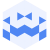Am 14. Feb. 2023 | 19:45 Uhr

# Feb 14 2023 | Tresor Linz | canceled but not canceled I Cochlear I dieb13Created at 17. Feb. 2023

217 Ansichten
##### by Tresor Linz

Tresor Linz - recorded on Feb. 14 2023

<<<<<<<<<<<<<<<<<<<<<<<<<<<<<<<<<<<<<<<<<<<<<<<<<<<<<<<<<
dieb13
<<<<<<<<<<<<<<<<<<<<<<<<<<<<<<<<<<<<<<<<<<<<<<<<<<<<<<<<<

Dieter Kovačič aka dieb13 gilt seit seinen Anfängen in den 1990er Jahren als Protagonist des Turntablism. Dafür dringt er nicht nur tief in die verrauschten, abstrakten Klänge des Vinyls vor, sondern fügt dem Klang auch Brüche hinzu und erzeugt durch sanfte Fadings und Überlagerungen eigenständige, beinahe animistische Soundscapes, die von einer Stimmung in die nächste gleiten. 2000 gründete Kovačič die Plattform www.klingt.org für experimentelle Musik aus Österreich. (Shilla Strelka) Link https://dieb13.klingt.org/

<<<<<<<<<<<<<<<<<<<<<<<<<<<<<<<<<<<<<<<<<<<<<<<<<<<<<<<<<
Cochlear
<<<<<<<<<<<<<<<<<<<<<<<<<<<<<<<<<<<<<<<<<<<<<<<<<<<<<<<<<
Synth -Noise und orientalischer Sirenen Gesang erzwingen die Gehörsschnecke nach außen kriechen zu wollen. Wirbel für Wirbel zerstören Schallwellen worauf menschliche Konventionen aufgebaut haben.

<<<<<<<<<<<<<<<<<<<<<<<<<<<<<<<<<<<<<<<<<<<<<<<<<<<<<<<<<
Tresor Linz
<<<<<<<<<<<<<<<<<<<<<<<<<<<<<<<<<<<<<<<<<<<<<<<<<<<<<<<<<

Raum für Klangkunst und künstlerische Experimente.

Space for sound art and artistic experiments.

##### Share & Embed
Embed this Video